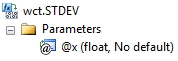# SQL Server standard deviation function

STDEV

Updated: 28 February 2011

Note: This documentation is for the SQL2008 (and later) version of this XLeratorDB function, it is not compatible with SQL Server 2005.

Use the aggregate function STDEV to return the estimated standard deviation based on a sample. This function differs from the built-in SQL Server STDEV function in that it employs a two-pass and more numerically stable method for calculating the standard deviation and under certain conditions will return a different value than the built-in function.

The standard deviation is a measure of how widely values are dispersed from the mean. The equation for STDEV is:SyntaxArguments
@x
the values used in the standard deviation calculation. @ x is an expression of type float or of a type that can be implicitly converted to float.
Return Types
float
Remarks
·         If you want measure the standard deviation for an entire population, then use the STDEVP function.
·         NULL values are not included in the standard deviation calculation.
·         STDEV is an aggregate function and follows the same conventions as all other aggregate functions in SQL Server.
·         If you have previously used the STDEV scalar function, the STDEV aggregate has a different syntax. The STDEV scalar function is no longer available in XLeratorDB/statistics2008, though you can still use the scalar STDEV _q.
Examples
SELECT wct.STDEV(x) as STDEV
FROM (VALUES
(91.3698),
(76.3382),
(74.5692),
(85.2957),
(99.0112),
(86.99),
(70.7837),
(72.834),
(78.1644),
(77.7472),
(66.0627),
(59.781),
(68.4793),
(78.6103),
(59.8621)
) n(x)

This produces the following result
STDEV
----------------------
11.0303511188038

(1 row(s) affected)

In the following example, we will use demonstrate the difference between the SQL Server STDEV calculation and XLeratorDB STDEV calculation. Using the XLeratorDB/math SeriesFloat function to create a million rows of randomly generated values between 1,000,000,000 and 1,000,000,010
SELECT ROUND(SeriesValue * 10, 0) + 1000000000 as x
INTO #n
FROM wctMath.wct.SERIESFLOAT(0,1,'',1000000,'R')

SELECT STDEV(x) as [SQL SERVER STDEV]
,wct.STDEV(x) as [XLDB STDEV]
,STDEV(x-1000000000) as [SQL SERVER STDEV adjusted]
FROM #n

DROP TABLE #n

This produces the following result. Your results wi
ll vary, since the dataset is randomly generated. The first column shows the built-in SQL Server STDEV calculation, the second shows the XLeratorDB calculation and the third column shows the built-in calculation for x minus 1,000,000,000.
SQL SERVER STDEV             XLDB STDEV SQL SERVER STDEV adjusted
---------------------- ---------------------- -------------------------
509.223830751183       2.91698668973923          2.91698668972115

(1 row(s) affected)

### SupportCopyright 2008-2023 Westclintech LLC         Privacy Policy        Terms of Service